# Class 10 RD Sharma Solution – Chapter 11 Constructions – Exercise 11.3

• Last Updated : 08 May, 2021

### Question 1. Draw a circle of radius 6 cm. From a point 10 cm away from its centre, construct the pair of tangents to the circle and measure their lengths.

Solution:

Follow these steps for construction :

Attention reader! All those who say programming isn't for kids, just haven't met the right mentors yet. Join the  Demo Class for First Step to Coding Coursespecifically designed for students of class 8 to 12.

The students will get to learn more about the world of programming in these free classes which will definitely help them in making a wise career choice in the future.

Step 1: Construct of 6 cm radius with centre O.

Step 2: Mark a point P, 10 cm away from the centre O.

Step 3: Now join PO then bisect it at M.

Step 4: From the centre M and the diameter PO, construct a circle that will be intersecting the given circle at T and S.

Step 5: Thus join PT and PS.

Further PT and PS are the required tangents.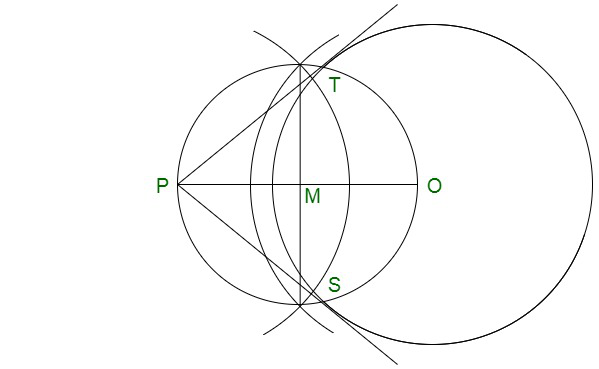### Question 2. Draw a circle of radius 3 cm. Take two points P and Q on one of its extended diameter each at a distance of 7 cm from its centre. Draw tangents to the circle from these two points P and Q.

Solution:

Follow these steps for construction :

Step 1: Construct of radius 3 cm with the centre O.

Step 2: Construct a diameter.

Step 3: Mark two points P and Q on this diameter with a distance of 7 cm each from the centre O, as shown below.

Step 4: Now bisect QO at N and PO at M.

Step 5: From the centres M and N, construct circle on ‘PO’ and ‘QO’ as diameter which intersect the given circle at S, T and S’, T’ respectively.

Step 6: Further join PS, PT, QS’ and QT’.

Therefore, PS, PT, QS’ and QT’ are the required tangents to the given circle.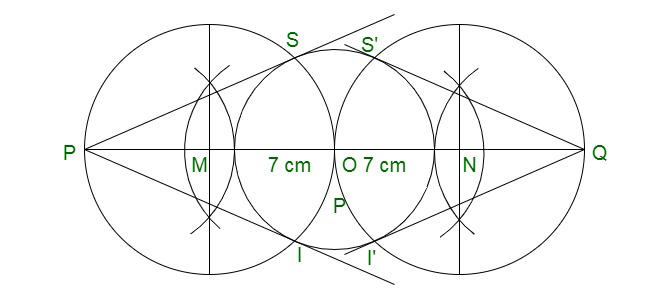### Question 3. Draw a line segment AB of length 8 cm. Taking A as centre, draw a circle of radius 4 cm and taking B as centre, draw another circle of radius 3 cm. Construct tangents to each circle from the centre of the other circle. [CBSE 2013]

Solution:

Step 1: Construct a line segment AB of 8 cm.

Step 2: Draw circles from the centre A and radius 4 cm and with centre B and radius 3 cm.

Step 3: Now bisect AB at M.

Step 4: From centre M and diameter AB, construct a circle which will intersects the two circles at S’, T’ and S, T respectively.

Step 5: Further join AS, AT, BS’and BT’.

Therefore, AS, AT, BS’ and BT’ are the required tangent.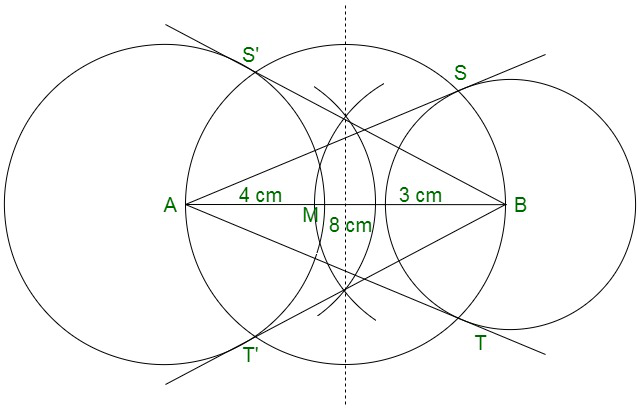### Question 4. Draw two tangents to a circle of radius 3.5 cm from a point P at a distance of 6.2 cm from its centre.

Solution:

Follow these steps for construction :

Step 1: Construct a circle of radius 3.5 cm with centre O.

Step 2: Mark a point P which will be of 6.2 cm from O.

Step 3: Now bisect PO at M and construct a circle with centre M and diameter OP which will intersects the given circle at T and S respectively.

Step 4: Further join PT and PS.

Therefore, PT and PS are the required tangents to circle.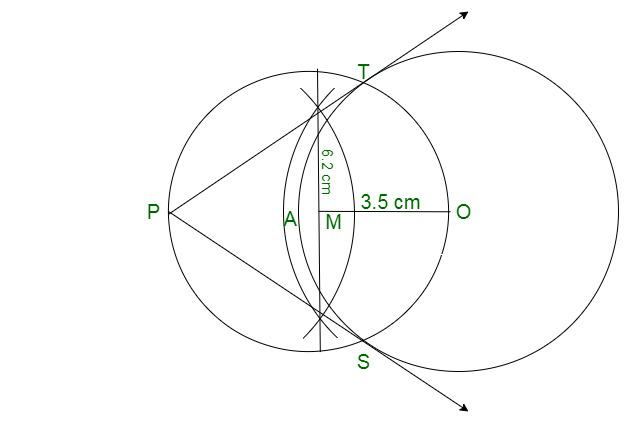### Question 5. Draw a pair of tangents to a circle of radius 4.5 cm, which are inclined to each other at an angle of 45°. [CBSE 2013]

Solution:

At centre the angle is 180° – 45° = 135°

Step 1: Construct a circle of radius 4.5 cm with centre O.

Step 2; Now, at O, construct an angle ∠TOS = 135°

Step 3: Further at T and S draw perpendicular which will meet at P.

Therefore, PT and PS are the tangents which inclined each other 45°.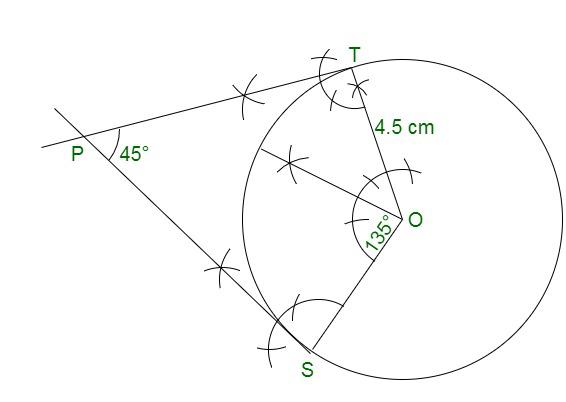### Question 6. Draw a right triangle ABC in which AB = 6 cm, BC = 8 cm and ∠B = 90°. Draw BD perpendicular from B on AC and draw a circle passing through the points B, C and D. Construct tangents from A to this circle.

Solution:

Step 1: Construct a line segment BC of 8 cm

Step 2: From B construct an angle of 90°

Step 3: Construct an arc BA˘ of 6cm cutting the angle at A.

Step 4: Now join AC. Thus, ΔABC is the required A.

Step 5: Construct perpendicular bisector of BC cutting BC at M.

Step 6: Mark M as centre and BM as radius, construct a circle.

Step 7: Mark A as centre and AB as radius, construct an arc cutting the circle at E. Thus, join AE.

Therefore, AB and AE are the required tangents.

Justification:

Given: ∠ABC = 90°

Thus, OB is a radius of the circle.

Therefore, AB is a tangent to the circle.

Also AE is a tangent to the circle.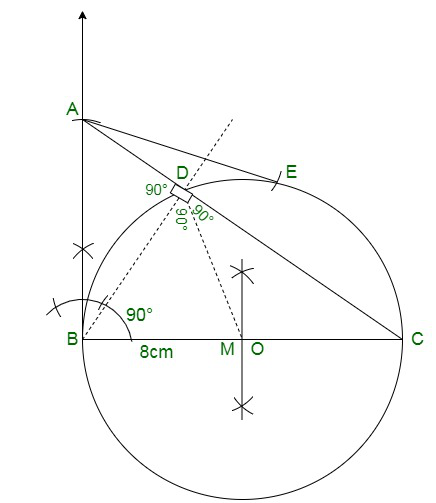### Question 7. Draw two concentric circles of radii 3 cm and 5 cm. Construct a tangent to the smaller circle from a point on the larger circle. Also, measure its length. [CBSE 2016]

Solution:

Given: Two concentric circles of radii 3 cm and 5 cm with centre O.

We have to construct a pair of tangents from point P on outer circle to the other.

Step 1: Construct two concentric circles of radii 3 cm and 5 cm with the centre O.

Step 2: Then, take any point P on outer circle and join OP.

Step 3: Now, bisect OP and let M’ be the mid-point of OP.

Take M’ as centre and OM’ as radius construct a circle dotted which will cut the inner circle as M and P’.

Step 4: Further, join PM and PP’. Therefore, PM and PP’ are the required tangents.

Step 5: After measuring PM and PP’, we find that PM = PP’ = 4 cm.

Actual calculation:

In right angle ΔOMP, ∠PMO = 90°

Therefore,

PM2 = OP2 – OM2   {by Pythagoras theorem i.e. (hypotenuse)2 = (base)2 + (perpendicular)2}

⇒ PM2 = (5)2 – (3)2 = 25 – 9 = 16

⇒ PM = 4 cm

Hence, the length of both tangents is 4 cm.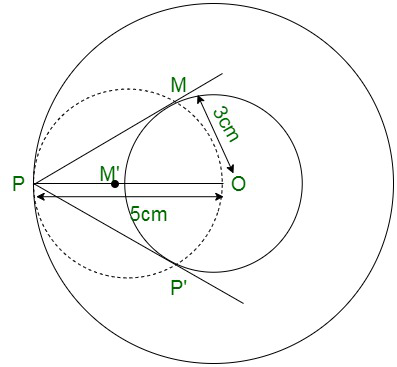My Personal Notes arrow_drop_up